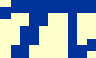1 A star has a measured V-band magnitude of 20.0. How many photons per second are detected from this star by a 4.2 m telescope with an overall telescope/instrument/filter/detector efficiency of 30%? The relation between fluxes and magnitudes is given by the equation: m1-m2 = -2.5 log10(F1/F2). We can see from table 1 that a V = 0 star has a monochromatic flux of Fλ = 3.61 x 10-11 W m-2 nm-1. Substituting these values into the equation above, we obtain: 20-0 = -2.5 log10(F1/3.61 x 10-11), which gives F1 = 3.61 x 10-19 W m-2 nm-1 at the effective wavelength of the V band, 550 nm. The number of photons s-1 m-2 nm-1 is then given by dividing this flux by the energy of a single 550 nm photon, i.e. N1 = F1 λ / h c = 1.0 photons s-1 m-2 nm-1. The total number of photons detected by a 4.2 m telescope in the V band can be obtained by multiplying this number by the collecting area of the telescope, the bandpass of the filter and the efficiency of the telescope/instrument/filter/detector: N1 = 1.0 xx 2.12 x 86 x 0.3 = 357 photons s-1.

 2 A non-variable star is tracked across the sky on a photometric night. At a zenith distance of 30o, 100,000 photons are detected from the star in a 1 minute integration in the V band, which drops to 88,000 photons at a zenith distance of 60o. What is the extinction coefficient in the V band on the night in question? You may assume that the signal from the sky has been subtracted from these measurements. The instrumental magnitude of the star at each zenith distance is given by minst = -2.5 log10 (F/texp), and these can be corrected for extinction using the equation: minst = minst0 + k sec z. Hence, we can write: minst1 = -2.5 log10 (100,000/60) = minst0 + k sec 30, and minst2 = -2.5 log10 (88,000/60) = minst0 + k sec 60. Subtracting these two equations to eliminate minst0 and rearranging for k, we obtain: k = 0.16 magnitudes per airmass in the V band.

 3 The star Vega is observed on the same night with the same equipment at a zenith distance of 45o. If 1 x 107 photons are detected from Vega in a 10 s exposure, what is the V-band magnitude of the star in question 2? The instrumental magnitude of Vega at a zenith distance of 45o is: minststd = -2.5 log10 (F/texp) = -2.5 log10 (1 x 107/10) = -15. The above-atmosphere instrumental magnitude of Vega is then given by: minst0std = minst - k sec z = -15 - 0.16 sec 45 = -15.2. In comparison, the above-atmosphere instrumental magnitude of the star in question 2 is: minst0 = minst1 - k sec z = -2.5 log10 (100,000/60) - 0.16 sec 30 = -8.2. Table 1 tells us that Vega has a magnitude of V = 0. Hence the zero point of the telescope/instrument/filter/detector combination is mzp = mstd - minst0std = 0 - (-15.2) = 15.2 The calibrated V-band magnitude of the star in question 2 is then given by: m = mzp + minst0 = 15.2 + (-8.2) = 7.

 4 Using the same equipment as described in question 1, if the sky has a brightness of V = 19.9 magnitudes per square arcsecond, and the extraction aperture has a radius of 2.5", how many photons per second from the sky are recorded in the aperture? The solution to this question follows the method used in question 1, but remembering that the sky is not a point source like a star. Hence, unlike a star, the larger the aperture used to measure the sky, the higher the total number of photons that will be detected from the sky. For this reason, sky magnitudes are quoted in per square arcsecond units and the area of the aperture used to extract the signal from the CCD image must be taken into account. The relation between fluxes and magnitudes is given by the equation: m1-m2 = -2.5 log10(F1/F2). We can see from table 1 that a V = 0 star has a monochromatic flux of Fλ = 3.61 x 10-11 W m-2 nm-1. Substituting these values into the equation above, we obtain: 19.9-0 = -2.5 log10(F1/3.61 x 10-11), which gives F1 = 3.96 x 10-19 W m-2 nm-1 arcsecond-2 from the sky at the effective wavelength of the V band, 550 nm. The number of photons s-1 m-2 nm-1 arcsecond-2 is then given by dividing this flux by the energy of a single 550 nm photon, i.e. N1 = F1 λ / h c = 1.1 photons s-1 m-2 nm-1 arcsecond-2. The total number of photons per second from the sky detected in the extraction aperture by a 4.2 m telescope in the V band can be obtained by multiplying the number above by the collecting area of the telescope, the bandpass of the filter, the efficiency of the telescope/instrument/filter/detector, and the area of the aperture: N1 = 1.1 x (x 2.12) x 86 x 0.3 x (x 2.52)= 7720 photons s-1.